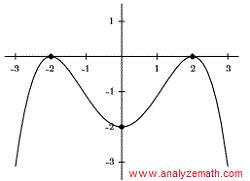# Find a Polynomial Given its Graph Questions with Solutions

How to find a polynomial given its graph? Questions are presented along with their detailed solutions and explanations.

## Question 1

Find the equation of the cubic polynomial function g shown below..

Solution
The graph of the function has one zero of multiplicity 1 at x = -1 which corresponds to the factor x + 1 and and a zero of multiplicity 2 at x = 3 (graph touches but do not cut the x axis) which corresponds to the factor (x - 3)2, hence function g has the equation:
g(x) = k (x + 1)(x - 3)2 , where k is a constant.
Constant k may be found using the point with coordinates (1 , 3) shown in the graph.
g(1) = k (1 + 1)(1 - 3)2 = 3
Simplify and solve for k.
k = 3 / 8
g(x) is given by.
g(x) = (3 / 8)(x + 1)(x - 3)2

## Question 2

Find the fourth-degree polynomial function f whose graph is shown in the figure below..

Solution
The graph of the polynomial has a zero of multiplicity 1 at x = 2 which corresponds to the factor (x - 2), another zero of multiplicity 1 at x = -2 which corresponds to the factor (x + 2), and a zero of multiplicity 2 at x = -1 (graph touches but do not cut the x axis) which corresponds to the factor (x + 1)2, hence the polynomial f has the equation:
f(x) = k (x - 2)(x + 2)(x + 1)2 , where k is a constant.
Constant k may be found using the y intercept f(0) = - 1 shown in the graph.
f(0) = k(0 - 2)(0 + 2)(0 + 1)2 = -1
Simplify and solve for k.
k = 1 / 4
f(x) is given by.
f(x) = (1/4)(x - 2)(x + 2)(x + 1)2

## Question 3

Find the equation of the degree 4 polynomial f graphed below..

Solution
The graph has x intercepts at x = 0 and x = 5 / 2. These x intercepts are the zeros of polynomial f(x). Because the graph crosses the x axis at x = 0 and x = 5 / 2, both zero have an odd multiplicity. The graph at x = 0 has an 'cubic' shape and therefore the zero at x = 0 has multiplicity of 3. The shape of the graph at x = 1/2 is close to linear hence the zero at x = 5 / 2 has multiplicity equal to 1. Using the zeros at x = 0 and x = 5 / 2, f(x) may be written as
f(x) = k (x - 0)3 (x - 5 / 2) , where k is a constant.
We now use the point (2 , -4) to fink k.
- 4 = k(2)3 (2 - 5 / 2) , solve for k: k = 1
The equation of polynomial f(x) is given by.
f(x) = x3 (x - 5 / 2)

## Question 4

The graph of a cubic polynomial $y = a x^3 + b x^2 +c x + d$ is shown below. Find the coefficients a, b, c and d..

Solution
The polynomial has degree 3. The graph of the polynomial has a zero of multiplicity 1 at x = -2 which corresponds to the factor x + 2 and a zero of multiplicity 2 at x = 1 which corresponds to the factor (x - 1)2. Hence the polynomial may be written as
y = k(x + 2)(x - 1)2
We now need to find k using the y -intercept (0 , 1) shown in the graph.
1 = k(0 + 2)(0 - 1)2 = 2 k
Solve for k.
k = 1 / 2
We now expand the polynomial, write it in standard form and identify the coefficient a, b, c and d.
y = (1 / 2)(x + 2)(x - 1)2 = 0.5 x3 - 1.5 x + 1
We now compare the expression of the polynomial found above to
y = a x3 + b x2 +c x + d
and obtain the values of the coefficients
a = 0.5 , b = 0 , c = -1.5 and d = 1

## Question 5

The graph of polynomial $y=a x^4+bx^3+c x^2+d x+e$ is shown below. Find the coefficients b, d and e..

Solution
The graph of the polynomial is symmetric with respect to the y axis and therefore the polynomial function given above must be an even function. The terms   b x3   and   d x   included in the given expression of the polynomial above are not even and therefore their coefficients are equal to 0. Hence
b = 0 , d = 0
and therefore y is given by
y = a x4 + c x2 + e
Coefficient e is found using the y intercept (0 , -2) from the graph.
-2 = a (0)4 + c (0)2 + e
e = -2